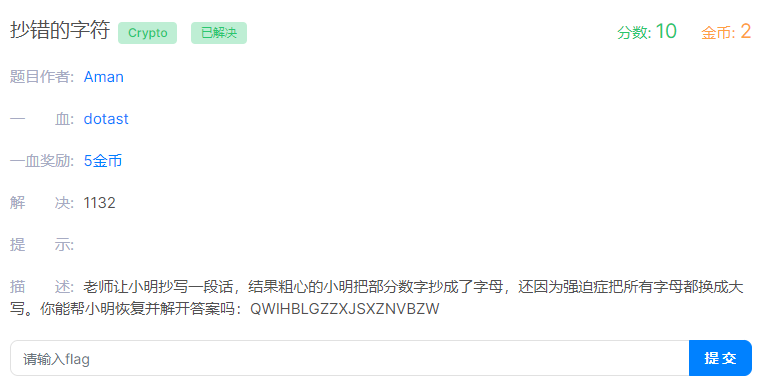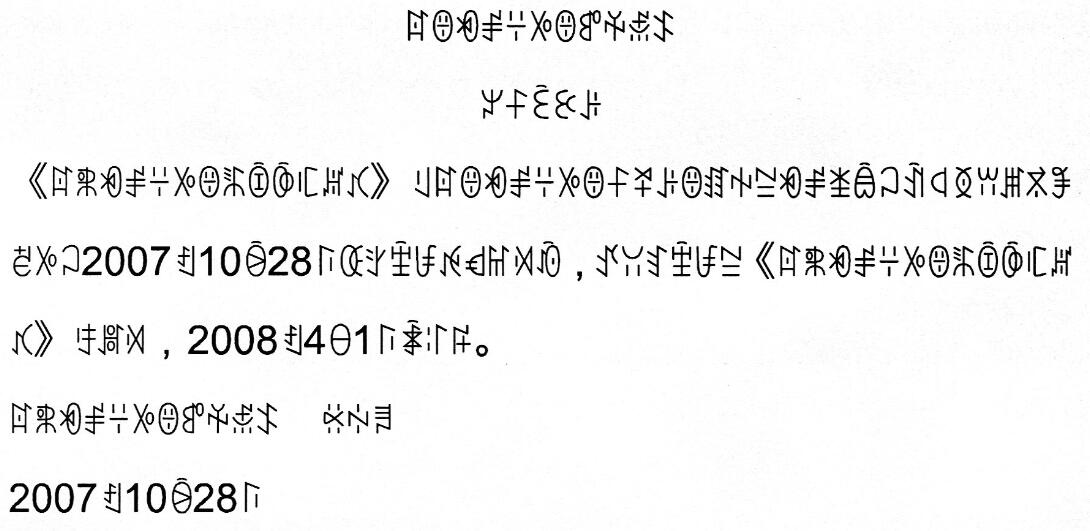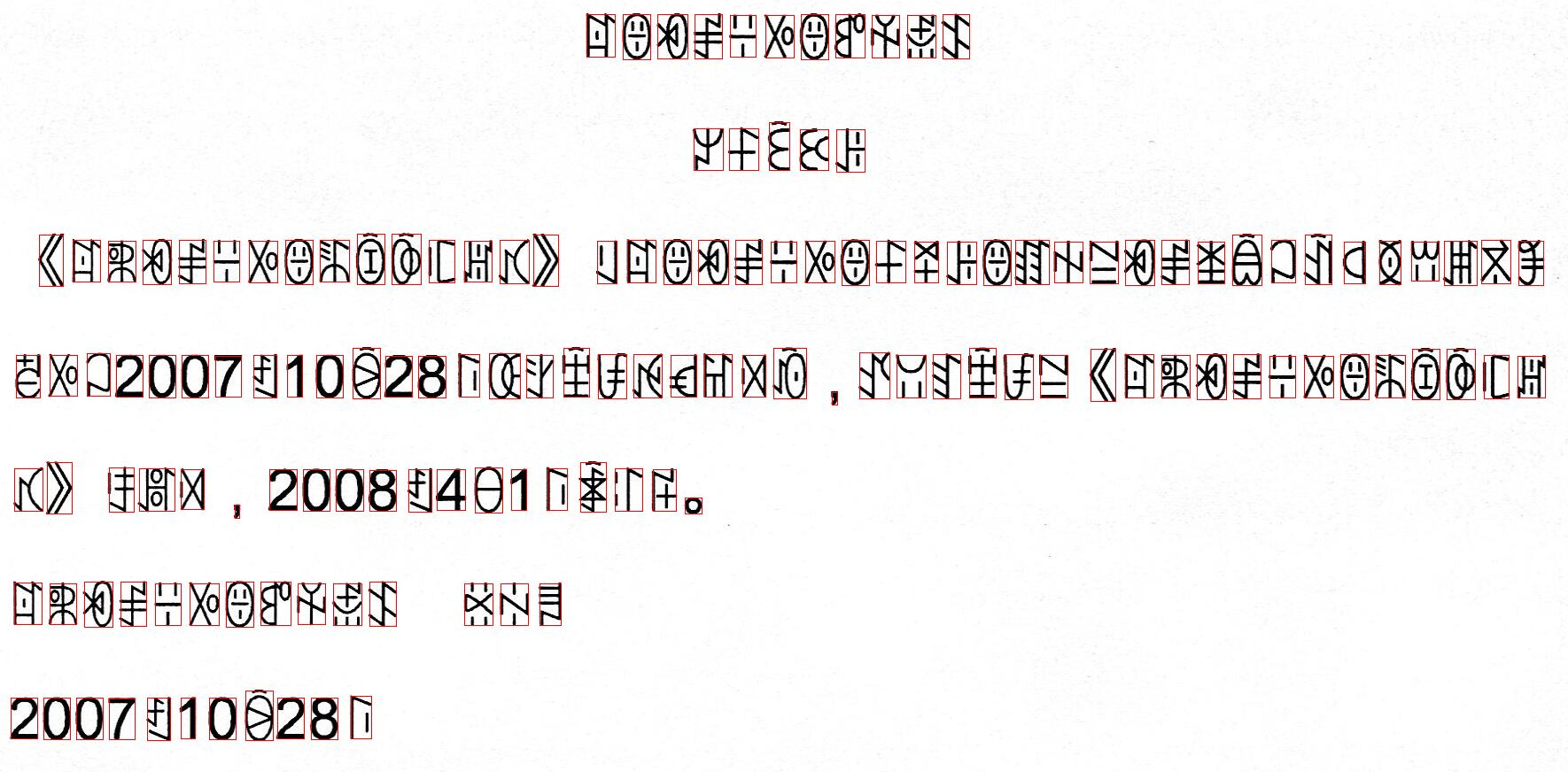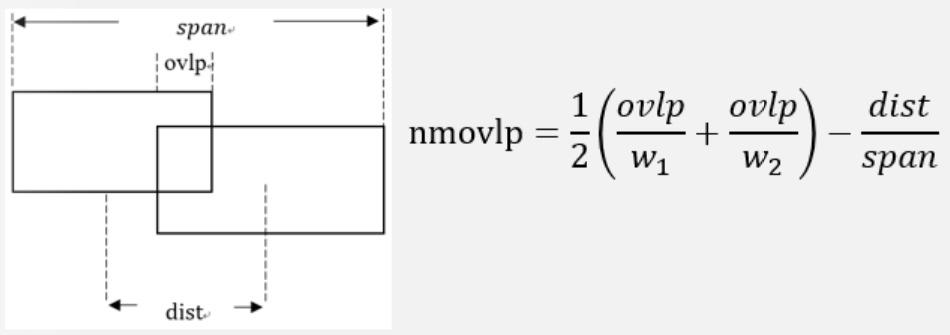• C语言，删除字符串中的指定字符，求找~
千次阅读
2021-05-19 07:54:42

你的错误就在于没有充分认识到c语言的字符串是以\0为结束符的

你的移动了字符后，没有在新字符传的尾部加上\0,

导致的结果是输出的数据后面出现有重复的字符。

你的算法存在不足是你出现这个问题的主要原因

你应该这样处理：

循环处理s1的所有字符，如果该字符出现在s2中，那么就不再处理

如果没有出现在s2中，就将该字符保存在第三个字符串中，

最后将第三个字符串的数据尾部增加\0后拷贝到s1中

这个效率要比你的算法效率高，不易出错

下面是实例代码：

/**

* 将字符串s1中的出现在s2中的字符全部删除，并在s1中返回

* @param s1

* @param s2

* @return

*/

char *rm(char *s1, char *s2) {

int iLen1;

int iLen2;

int flag;

int i, j, k;

char *tmp;

char ch;

if ((s1 == NULL) || (s2 == NULL)) {

//字符串有问题

return s1;

}

iLen1 = strlen(s1);

iLen2 = strlen(s2);

//申请临时空间，注意最后要有\0,因此多申请一个

tmp = (char*) malloc((iLen1 + 1) * sizeof (char));

if (tmp == NULL) {

//申请空间失败，没有办法处理了，只好返回NULL

return NULL;

}

k = 0;

for (i = 0; i < iLen1; i++) {

ch = s1[i];

flag = 0;

for (j = 0; j < iLen2; j++) {

if (s2[j] == ch) {

//该字符在s2中出现，不做任何处理

flag = 1;

break;

}

}

if (0 == flag) {

//该字符没有出现在s2中，加入到临时空间中

tmp[k] = ch;

k++;

}

}

//设置结束标志

tmp[k] = '\0';

strcpy(s1, tmp); //拷贝到目标传中

free(tmp); //释放申请的临时空间

return s1;

}

下面是数组的：

/**

* 将字符串s1中的出现在s2中的字符全部删除，并在s1中返回

* @param s1

* @param s2

* @return

*/

char *rm(char s1[], char s2[]) {

int iLen1;

int iLen2;

int flag;

int i, j, k;

char ch;

if ((s1 == NULL) || (s2 == NULL)) {

//字符串有问题

return s1;

}

iLen1 = strlen(s1);

iLen2 = strlen(s2);

k = 0;

for (i = 0; i < iLen1; i++) {

ch = s1[i];

flag = 0;

for (j = 0; j < iLen2; j++) {

if (s2[j] == ch) {

//该字符在s2中出现，不做任何处理

flag = 1;

break;

}

}

if (0 == flag) {

//该字符没有出现在s2中

if (i != k) {

//以前已经删除了一些字符，导致需要调整字符位置了

s1[k] = ch;

}

k++;

}

}

//设置结束标志

s1[k] = 0;

return s1;

}。

全部

更多相关内容
• 该文档包含了箭头类、基本形状类、货币类、数学类、音乐符号类、对错、星星、星座、象棋、扑克、希腊字母类、法律符号、标点和符号等特俗字符代码对照
• 为什么编码规范里要求每行代码不超过80个字符的限制是合理的。也许在Python编码风格指导(PEP8)中最有争议的一部分要数每行代码不超过80个字符的限制。没，实际上是79个字符，但我使用80个字符，这个大概数，它是给...规范
• Bugku CTF 抄字符 WP 题目 思路： 由描述知给出的字符串中的每个字符原来可能是大小写字母或数字，应该先暴力枚举，并且优先级：数字 > 小写字母 > 大写字母，然后将枚举出来的字符进行解密（根据评论区...

题目思路：
由描述知给出的字符串中的每个字符原来可能是大小写字母或数字，应该先暴力枚举，并且优先级：数字 > 小写字母 > 大写字母，然后将枚举出来的字符进行解密（根据评论区知道是base64,实际做题过程中可能就需要一个一个尝试了）
ps:字符串最后还要加一个=是真的搞

代码如下：

import base64
s = "QWIHBLGZZXJSXZNVBZW="
dic = {'I': '1', 'B': '6', 'S':'5','G': '9','Z':'2'}
res = ''

def check(s):
f = True
s = str(s)
for i in range(len(s)):
if ((s[i] in list('_+=')) or s[i].isdigit() or s[i].isalpha()):
continue
else:
f = False
break
return f

def strcon_decode(s,i):
global res
if(i==4):
ss = ''.join(s)
sss = base64.b64decode(ss)
sss = str(sss)[2:-1]
if check(sss):
# print(ss+' decode: ' + sss)
ss = res + sss
res = ss
return True
else:
return False
else:
if s[i] in dic.keys():
ss = s[i]
s[i] = dic[s[i]]
f = strcon_decode(s,i+1)
s[i] = ss
if f:
return True
s[i] = s[i].lower()
f = strcon_decode(s,i+1)
s[i] = s[i].upper()
if f:
return f
return strcon_decode(s,i+1)

for i in range((len(s)//4)):
ss = s[i*4:(i+1)*4]
#     print(ss+' decode: ')
ss = list(ss)
strcon_decode(ss,0)

print(res)


最后记得还要写成flag{res}的形式

CTF萌新的第一篇WP

展开全文网络安全
• ## Python20行代码实现视频字符化

万次阅读 多人点赞 2020-04-11 15:24:10
Python20行代码实现视频字符化 我们经常在B站上看到一些字符鬼畜视频，主要就是将一个视频转换成字符的样子展现出来。看起来是非常高端，但是实际实现起来确是非常简单，我们只需要接触opencv模块，就能很快的实现...

# Python20行代码实现视频字符化

我们经常在B站上看到一些字符鬼畜视频，主要就是将一个视频转换成字符的样子展现出来。看起来是非常高端，但是实际实现起来确是非常简单，我们只需要接触opencv模块，就能很快的实现视频字符化。但是在此之前，我们先看看我们实现的效果是怎样的：上面就是截取的一部分效果图，下面开始进入我们的主题。

## 一、OpenCV的安装及图片读取

在Python中我们只需要用pip安装即可，我们在控制台执行下列语句：

pip install opencv-python


安装完成就可以开始使用。我们先读取一个图片：

import cv2
cv2.imshow('im', im)	# 显示图片
cv2.waitKey(0)	# 等待键盘输入
cv2.destroyAllWindows()	# 销毁内存


首先我们使用cv2.imread方法读取图片，该方法返回一个ndarray对象。然后调用imshow方法显示图像，调用后会出现一个窗口，因为这个窗口只会出现一瞬间，所以我们调用waitKey等待输入，传入0表示无限等待。因为opencv是使用c++编写的，所以我们需要销毁内存。

## 二、OpenCV中的一些基础操作

我们将视频字符化的思路就是先将视频转换为一帧一帧的图像，然后对图像进行字符化处理，最后展示出来就是字符视频的效果了。在我们生成字符画之前，我们还要看一些OpenCV的操作。

### （1）灰度转换

灰度处理是一个非常常用的操作，我们原始的图片是有BGR三个图层（在OpenCV中，图像是以BGR形式读取）。我们进行灰度处理直观上看就是将图片变成黑白，而本质上是将图片的三个图层通过计算，变成一个图层。而这种计算是不需要我们做的，我们只需要调用OpenCV中的函数即可：

import cv2
# 读取图片
# 灰度转换
grey = cv2.cvtColor(frame, cv2.COLOR_RGB2GRAY)


效果图和原图对比如下：左边为原图，右边为灰度转换后的图像。

### （3）改变图片大小

因为字符化后图像会比较大，所以我们需要先缩小图片，我们调用cv2.resize即可改变图像大小：

import cv2
# 读取图像
# 改变图像大小
re = cv2.resize(im, (100, 40))
cv2.imshow('11', re)
cv2.waitKey(0)
cv2.destroyAllWindows()


### （2）逐帧读取视频

我们可以通过VideoCapture读取视频，然后调用其中的方法读取每一帧。

import cv2
# 读取视频
video = cv2.VideoCapture('jljt.mp4')
# 读取帧，该方法返回两个参数，第一个为是否还有下一帧，第二个为帧的ndarray对象
while ret:
# 循环读取帧


有了上面的操作，我们就可以开始我们下一步的工作了。

## 三、图片字符化

对于只有一个通道的图片，我们可以把它当成一个矩形，这个矩形最小单位就是一个像素。而字符化的过程就是用字符替代像素点的过程。所以我们要遍历图像的每个像素点，但是我们应该用什么字符取代呢？

我们颜色有一个参照表，而opencv将这个参数表切割成256份，代表不同的程度，我们也可以做一个参照表，不过表中的内容不是颜色，而是字符。上图为颜色表，我们可以使颜色表和字符表建立映射关系。假如字符表如下：

mqpka89045321@#$%^&*()_=||||} 我们可以得到下列公式：经过变换可以求得相应颜色对应字符表中的字符：这个公式不理解也没关系，只需要会用即可。下面就是我们完整的代码了： import cv2 str = 'mqpka89045321@#$%^&*()_=||||}' # 字符表
grey = cv2.cvtColor(im, cv2.COLOR_BGR2GRAY)	# 灰度转换
grey = cv2.resize(grey, (50, 18))	# 缩小图像
str_img = ''	# 用于装字符画
for i in grey:	# 遍历每个像素
for j in i:
index = int(j / 256 * len(str))	# 获取字符坐标
str_img += str[index]	# 将字符添加到字符画中
str_img += '\n'
print(str_img)


生成如下字符画：因为尺寸比较小的关系，看出来的效果不是很好，我们调节好大小就好了。

## 四、视频转字符

我们知道图片转字符，自然视频转字符就不是什么问题了，我们只需要在逐帧读取中执行图片字符化操作即可。

import os
import cv2
str = 'mqpka89045321@#$%^&*()_=||||}' # 字符表 video = cv2.VideoCapture('jljt.mp4') # 读取视频 ret, frame = video.read() # 读取帧 while ret: # 逐帧读取 str_img = '' # 字符画 grey = cv2.cvtColor(frame, cv2.COLOR_RGB2GRAY) # 灰度转换 grey = cv2.resize(grey, (100, 40)) # 该表大小 for i in grey: # 遍历每个像素点 for j in i: index = int(j / 256 * len(str)) # 获取字符坐标 str_img += str[index] # 将字符添加到字符画中 str_img += '\n' os.system('cls') # 清除上一帧输出的内容 print(str_img) # 输出字符画 ret, frame = video.read() # 读取下一帧 cv2.waitKey(5)  这样我们就会每个5毫秒执行一帧画面，在我们使用pycharm执行时，会发现并没有执行清屏操作，所以我们需要到命令行运行。最终效果就是我们的字符视频了：在选取字符表时我们需要注意主体的颜色，如果主体颜色较浅，则字符表的尾部应该为一些复杂字符，如：$%#@&。字符表头部为一些简单字符，如：-|/等。如果主体颜色较深，而背景颜色较浅，则反之。当然这没有唯一的标准，大家可以慢慢调节。感兴趣的读者，可以关注我的个人公众号：ZackSock，看到抠鼻屎的就是我没错了。

展开全文• 由于印刷体版式工整，加之彝语的结构较为简单，遂采取了单字符分割+识别的流程来进行彝语的识别工作。（其实主要还是因为训练样本不够啊，外加“某局”希望能得到单字符的坐标，不然直接用CRNN来做序列识别可能会更...

之前一段时间做了一个少数民族语言的文字检测与识别的项目，一共有6种语言，其中的彝语的识别相对简单，部分彝语文档图片如下。由于印刷体版式工整，加之彝语的结构较为简单，遂采取了单字符分割+识别（此算法同样可适用于汉字韩语日语等类似结构文字的单字符分割部分）的流程来进行彝语的识别工作。（其实主要还是因为训练样本不够啊，外加甲方希望能得到单字符的坐标，不然直接用CRNN来做序列识别可能会更简单一点，卒。。。）上图所示为彝语打印文档的一张扫描件示例图。由图可见彝语的结构跟汉字在某些程度上有些相似之处，比如都属于方块字，存在上下结构和左右结构等。由图可发现彝语的识别可以采用单字符分割+识别的方式来进行处理。下面是我对这部分（单字符分割）的处理代码。（此代码经修改后同样可适用于汉字韩语日语等类似结构文字的单字符分割部分）

import cv2    # 版本为3.3.10，安装4.0及以上版本可能会不兼容
import os
import numpy as np

def display(img):
cv2.imshow("1", img)
cv2.waitKey(0)

def get_split_line(img, projection_row):
split_line_list = []
flag = False
start = 0
end = 0
for i in range(0, len(projection_row)):
if flag == False and projection_row[i] > 0:
flag = True
start = i
elif flag and (projection_row[i] == 0 or i == len(projection_row) - 1):
flag = False
end = i
if end - start < 15:  # need specify or rewrite
flag = True
continue
else:
split_line_list.append((start, end))
return split_line_list

def get_contours(img):
contour_list = []
contours = cv2.findContours(img, cv2.RETR_EXTERNAL, cv2.CHAIN_APPROX_SIMPLE)
for i in range(0, len(contours)):
x, y, w, h = cv2.boundingRect(contours[i])
contour_list.append((x, y, w, h))
# cv2.rectangle(img_input, (x,y), (x+w, y+h), (0,0,255))
return contour_list

def sort_merge(contour_row):
contour_row = sorted(contour_row, key=lambda x: x)  # sort by x
# print(contour_row)
i = 0
for _ in contour_row:    # 这部分的合并规则用的是刘成林老师paper中的方法
if i == len(contour_row) - 1 or contour_row[i] == -1:
break
# print(contour_row[i])
rectR = contour_row[i + 1]
rectL = contour_row[i]
ovlp = rectL + rectL - rectR
dist = abs((rectR + rectR / 2) - (rectL - rectL / 2))
w_L = rectL + rectL
w_R = rectR + rectR
span = (w_R if w_R > w_L else w_L) - rectL
nmovlp = (ovlp / rectL + ovlp / rectR) / 2 - dist / span / 8
if nmovlp > 0:
x = rectL
y = (rectL if rectL < rectR else rectR)
w_L = rectL + rectL
w_R = rectR + rectR
w = (w_R if w_R > w_L else w_L) - x
h_L = rectL + rectL
h_R = rectR + rectR
h = (h_R if h_R > h_L else h_L) - y
contour_row[i] = (x, y, w, h)
contour_row.pop(i + 1)  # after pop , index at i
contour_row.append((-1, -1, -1, -1))  # add to fix bug(the better way is use iterator)
i -= 1
i += 1
# print(contour_row)
return contour_row

def combine_verticalLine(contour_row):
i = 0
pop_num = 0
for _ in contour_row:
rect = contour_row[i]
if rect == -1:
break

if rect == 0:
i += 1
continue

if rect * 1.0 / rect > 4:
if i != 0 and i != len(contour_row) - 1:
rect_left = contour_row[i - 1]
rect_right = contour_row[i + 1]
left_dis = rect - rect_left - rect_left
right_dis = rect_right - rect - rect
# if left_dis <= right_dis:
if left_dis <= right_dis and rect_left < rect_right:
x = rect_left
y = (rect_left if rect_left < rect else rect)
w = rect + rect - rect_left
h_1 = rect_left + rect_left
h_2 = rect + rect
h_ = (h_1 if h_1 > h_2 else h_2)
h = h_ - y
contour_row[i - 1] = (x, y, w, h)
contour_row.pop(i)
contour_row.append((-1, -1, -1, -1))
pop_num += 1
# don't need recursive merge, causing it's left and right merge
else:
x = rect
y = (rect if rect < rect_right else rect_right)
w = rect_right + rect_right - rect
h_1 = rect_right + rect_right
h_2 = rect + rect
h_ = (h_1 if h_1 > h_2 else h_2)
h = h_ - y
contour_row[i] = (x, y, w, h)
contour_row.pop(i + 1)
contour_row.append((-1, -1, -1, -1))
pop_num += 1
i += 1
for i in range(0, pop_num):
contour_row.pop()
return contour_row

def split_oversizeWidth(contour_row):
i = 0
for _ in contour_row:
rect = contour_row[i]
if rect * 1.0 / rect > 1.2:  # height/width>1.2 -> split
x_new = int(rect + rect / 2 + 1)
y_new = rect
w_new = rect + rect - x_new
h_new = rect
contour_row[i] = (rect, rect, int(rect / 2), rect)
contour_row.insert(i + 1, (x_new, y_new, w_new, h_new))
i += 1
return contour_row

def image_preprocess(img_input):
gray_img = cv2.cvtColor(img_input, cv2.COLOR_BGR2GRAY)
gray_img = cv2.GaussianBlur(gray_img, (3, 3), 3)
_, img = cv2.threshold(gray_img, 0, 255, cv2.THRESH_OTSU)  # 将一幅灰度图二值化 input-one channel

_, img = cv2.threshold(img, 0, 255, cv2.THRESH_BINARY_INV)
kernel = cv2.getStructuringElement(cv2.MORPH_CROSS, (3, 3))
img = cv2.erode(img, kernel)
# height,width=img.shape[:2]
# img=cv2.resize(img,(int(width/2),int(height/2)),interpolation=cv2.INTER_CUBIC)
# display(img)
return img

def get_segmentation_result(img):  # has been eroded
projection_row = cv2.reduce(img, 1, cv2.REDUCE_SUM, dtype=cv2.CV_32S)  # projection
split_line_list = get_split_line(img, projection_row)  # split image as row
segmentation_result = []
for i in split_line_list:
img_row = img[i:i, :]
contour_row = get_contours(img_row)
contour_row = sort_merge(contour_row)
contour_row = split_oversizeWidth(contour_row)
contour_row = combine_verticalLine(contour_row)
segmentation_result.append(contour_row)
for (x, y, w, h) in contour_row:  # draw
y += i
cv2.rectangle(img_input, (x, y), (x + w, y + h), (0, 0, 255))
# cv2.imwrite("./test1/n003.jpg",img_input)
return segmentation_result

pic_path = './img003.jpg'

img_input = cv2.imread(pic_path, 1)  # (2975, 1787, 3)   但是图片查看器显示的是  1787 * 2975
img = image_preprocess(img_input)  # erode
segmentation_result = get_segmentation_result(img)  # store segmentation result : [(x,y,w,h),(),...]

# cv2.imwrite("./save.jpg", img_input)
display(img_input)


代码运行结果如下：此代码经过一定的修改可适用于很多的场景，大家有需要可以尝试一下。

下面大致说一下代码的流程（关注get_segmentation_result部分），字符分割的代码部分主要是采用“投影+连通域”的方式来实现。首先对图像进行二值化与腐蚀（断开印刷过程中可能存在的粘连）的操作，之后对预处理后的图片进行水平投影，得到图片中所有的文本行。然后分别对图片中的每一个文本行进行一系列操作，得到其连通域，然后再对连通域进行合并（合并规则是刘成林老师的一篇paper中的方法，代码中已经注释，具体操作步骤见下图），针对合并错的部分进行切分操作（针对过宽的部分），最后再对没有合并的部分高宽比很大的竖形字符进行单独的合并。进行完上述操作之后，就可以得到不错的分割效果。（具体流程和操作步骤仅供参考）有问题欢迎多交流，共同进步~

展开全文• 转义字符，顾名思义，首先，它是一个字符，其次它的意义转变了，但它还是yi'ge'zi'fu
• ## KMP —— 字符串分析算法

千次阅读 多人点赞 2020-11-30 07:34:01
在这个过程中我们找到了 3 前后缀： A 和 A ABA 和 ABA ABABA 和 ABABA 这三前后缀都是完全匹配的，但是第三是一个无效的前后缀，因为它的长度与我们这串字符的长度是一样的。那么就意味着我们完全不需要移动...算法 javascript 前端
• 5 道题征集代码界前 3% 的超级王者》——看到这个标题，我内心非常非常激动，因为终于可以证明自己技术很牛逼了。 但遗憾的是，凭借 8 年的 Java 开发经验，我发现这五道题自己全解了！惨痛的教训再次证明，我是那...Java
• 在h处前欲添加字符a时出现以下情况： 解决办法：按一下电脑键盘上的lnsert键即可解决。插入 覆盖
• R读数据出现的问题： 1. .xls文件变成.csv文件是不能单纯的更改扩展名，需要另存为.csv文件 2. ...Error in make.names(col.names, unique = TRUE) : 多字节字符串1有 此外: Warning messa...
• 三、字符串判断 [ -z STRING ] 如果STRING的长度为零则为真 ，即判断是否为空，空即是真； [ -n STRING ] 如果STRING的长度非零则为真 ，即判断是否为非空，非空即是真； [ STRING1 == STRING2 ] 如果两个字符串相同...
• 利用pillow模块处理，用20多行Python代码将普通图片转为字符画操作 这里是目录哟像素RGB值灰度值计算灰度值映射字符函数图片导入及尺寸设置遍历图片获取字符字符画输出和导入文件 哈喽，努力赚钱买生发水的大灰狼又...python 列表 机器学习 经验分享
• 字符串到常量池，一文看懂String类设计 从一道面试题开始 看到这个标题，你肯定以为我又要讲这道面试题了 // 这行代码创建了几个对象？ String s3 = new String("1"); 是的，没，我确实要从这里开始 这道题...常量池 运行时常量池
• 错误提示：该文件包含不能在当前代码页(936)中表示的字符。 错误提示：该文件含有xxx换行符 解决办法：1、点击错误提示，打开报错的文件。2、用鼠标左键单击一下该报文件位于编辑区的文件标签卡，以mainwindow...换行符
• 8可按字符串、16进制字节、文件方式进行发送，字符串和16进制字节可分别进行存储，内容互不干扰 9 按16进制发送时，可自动校验格式，不会输 10 可清空发送或接收区域的数据 欢迎c#有兴趣的爱好者们，加入c#技术...
• C++ 字符串 对象 C++ 字符串 对象 创建方法 C++ 字符串 对象 输出到控制台 C++ 字符串 拼接 C++ 字符串 方法调用 ( 栈内存对象 ) C++ 字符串 方法调用 ( 堆内存对象 ) ...C / C++ 字符串 完整代码示例
• 字符串格式不是很完美，导致JSON.parse转换失败。JSON.parse()方法对字符串的格式要求极其严格。一点格式错误都不能有，否则就报异常。 用eval或者\$parseJSON来解决json JSON.parse eval
• ## 中文字符导致出错

千次阅读 2021-04-26 21:53:29
把中文的一些字符，比如：逗号：，引号单引号左单引号：‘右单引号：’双引号左双引号：“右双引号”：”括号圆括号左圆括号：(右圆括号：)不小心写到了代码里，变成了代码的一部分，导致语法上报，导致代码无法...
• 让我判断测试用例的字符串中是否存在重复的字母，不论连续或是不连续，如果存在则返回false，不存在则返回true。是个经典问题。 首先冒出的想法就是循环遍历呗，用两重for循环，一层i=0,第二层i=i+1，然后用string....java
• 本篇文章通过我和三妹对话的形式来谈一谈“Unicode字符集简介”。 教妹学 Java，没见过这么有趣的标题吧？“语不惊人死不休”，没，本篇文章的标题就是这么酷炫，接受不了的同学就别点进来看了，所谓好奇心害死猫...unicode java
• 在Python中，既可以用单引号，也可以用双引号创建字符串。答：√狭义的包括以外币表示的 ____答：银行存款凭证 汇票 本票 支票中国大学MOOC: ...( )答：合法的字符常量是 _____。答：‘W’产品心理定价策略主要有_...
• 字符串“PAYPALISHIRING”在给定的行数上以锯齿形模式写入，如下所示：（您可能希望以固定字体显示此模式，以提高可读性）P A H NA P L S I I GY I R然后一行一行地读：“pahnaplshigyir”编写将接受字符串的代码，...
• 在运行Java代码时，可能会遇见如下图所示的错误：错误: 非法字符: ‘\ufeff’ 问题原因： 文件编码不一致，使用了UTF-8-BOM编码 解决办法： 用Notepad++打开该文件，点击工具类上的编码，选择“以UTF-8编码” 或者 ...
• 1、通过字符串名称 2、通过整数“HashID” private int speedID=Animator.StringTohash("Speed");  释义：从字符串“Speed”生成一个参数ID—“speedID”，ID用于参数的存储器优化（setters和getters...
• Java日期与字符串、时间戳相互转换前言日期转字符代码示例Date、Calender转字符串LocalDateTime、LocalDate转字符字符串转日期代码示例字符串转Date、Calender字符串转LocalDateTime、LocalDate日期转时间戳、...java
• 前文浏览器插件实现GitHub代码翻译原型演示之后, 意识到现有在线翻译服务已经具备部分设想的功能. 第一个测试, 选了一个很小的Python入门例程: class Person: pass # An empty block p = Person() print(p) ...机器翻译
• 编写代码时，光标变成了选择字符，而不是竖线 问题：今晚写代码时，某个关键字写了，要删除修改的时候，移动光标，竖线不是在两个字母中间了，而是覆盖了一个字母，这样我想要删除错误字母时，就会删除前面一个...windows
• 输入一个字符串，分别统计出其中英文字母、空格、数字和其它字符的个数，本文...若有符合条件的字符直接在类别数上加1就可以初始代码：# -*- coding:utf-8 -*-letter=0number=0space=0symbol=0print "请输入字符串：......